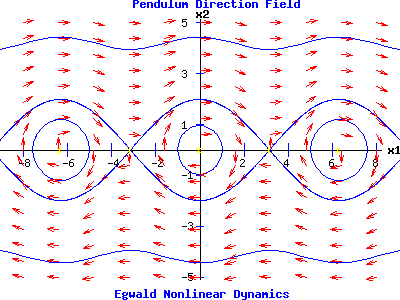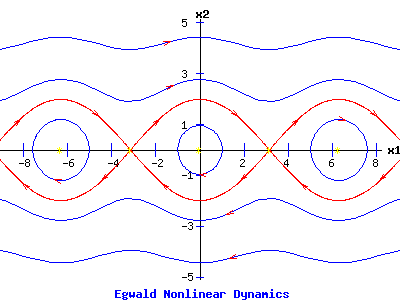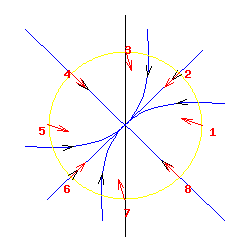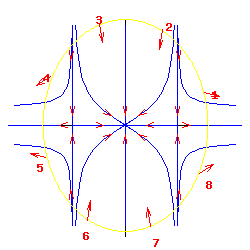Egwald Mathematics: Nonlinear Dynamics:

Mathematical Appendix

by

Elmer G. Wiens

Egwald's popular web pages are provided without cost to users.
Please show your support by joining Egwald Web Services as a Facebook Fan:Follow Elmer Wiens on Twitter:Introduction

Describe the dynamics of a two dimensional, continuous time process by a pair of differential equations and initial conditions:

dx1/dt = f1(x1, x2),         (1)

dx2/dt = f2(x1, x2),         (2)

or using vector notation:

xT = (x1, x2),     fT( x ) = ( f1( x ),  f2( x ) ), or

d x / dt = f( x ),

xT(0) = (x01,   x02).

where "T" indicates the transpose of a column vector to a row vector.

Unique solution trajectories, xT(t) = ( x1(t),  x2(t) ) = ( x1(t, x01),  x2(t, x02) ), evolve from each initial point.

System Flow

The flow of the system, Φt, is the set of all solution trajectories, defined by:

Φt( x(t) ) = { ( x1(t, x01), x2(t, x02) )  |  (x01, x02) is a legitimate initial point }.

Direction Field and Phase Portrait

A phase portrait provides qualitative information about the solutions to a system of differential equations, i.e. the system flow. Consider the system with equations:

dx1 / dt = f1(x1, x2) = x2,

dx2 / dt = f2(x1, x2) = -sin(x1),

describing the motion of a simple pendulum.

At any point (x1, x2) in the domain of this system, compute the vector ( f1(x1, x2),  f2(x1, x2) ) = ( x2,  -sin(x1) ). This vector indicates the direction in which the solution trajectory ( x1(t),  x2(t) ) passing through the point (x1, x2) travels. By plotting these (normalized) vectors at a lattice of points in the (x1, x2) space—called the direction field—one obtains a portrait of how solutions trajectories evolve over time.

The direction field of the system flow, and representative solution trajectories of the simple pendulum appear in the next diagram:Fixed Points

The fixed points of the nonlinear system are numbers x1* and x2* such that:

f1(x1*, x2*) = 0, and     (3)

f2(x1*, x2*) = 0,           (4)

Fixed points are also called equilibrium points of the system.

Nullclines

The locus of points (x1, x2) that satisfy equation (3) is called the x1-nullcline. The locus of points (x1, x2) that satisfy equation (4) is called the x2-nullcline.

Fixed points occur at the intersection(s) of the x1-nullcline and the x2-nullcline.

Stability of Fixed Points (Lorenz 31-34)

Nonlinear dynamical systems can have multiple fixed points which may be stable and/or unstable, requiring the distinction between local and global stability. Stable fixed points are called attractors, while unstable fixed points are called repellers.

Let the point x* be a fixed point, i.e. f( x* ) = 0.

Stable fixed point

• The fixed point is locally stable (locally Liapunov stable) if for every ε > 0 there exists a δ > 0 such that:

| x(0) - x* |  ≤  δ  →  | Φt(x(0)) - x* |  ≤  ε for all t.

• The fixed point is asymptotically stable if it is locally stable and if there exists a δ > 0 such that:

| x(0) - x* |  ≤  δ  →  lim t → ∞ | Φt(x(0)) - x* | = 0.

• The fixed point is globally asymptotically stable if it is locally stable and if :

lim t → ∞ | Φt(x(0)) - x* | = 0 for every legitimate x(0).

Linearization at a Fixed Point

To examine the solution trajectories of the nonlinear system:

dx / dt = f(x, y),

dy / dt = g(x, y),

near the fixed point (x*, y*), use the Taylor series expansions of the functions f and g about (x*, y*):

f(x, y) = f(x*, y*) + (x - x*)* fx(x*, y*) + (y - y*) * fy(x*, y*)
+ (1/2)*(x - x*)2 * fx,x(x*, y*) + (x - x*) * (y - y*) * fx,y(x*, y*) + (1/2)*(y - y*)2 * fy,y(x*, y*) + ...

g(x, y) = g(x*, y*) + (x - x*)* gx(x*, y*) + (y - y*) * gy(x*, y*)
+ (1/2)*(x - x*)2 * gx,x(x*, y*) + (x - x*) * (y - y*) * gx,y(x*, y*) + (1/2)*(y - y*)2 * gy,y(x*, y*) + ...

If |x - x*| and |y - y*| are sufficiently small numbers, the terms involving fx,x, fx,y,, and fy,y are very small compared with the terms involving fx and fy. Since

f(x*, y*) = 0, and

g(x*, y*) = 0,

one can approximate the nonlinear system near the fixed point, (x*, y*), by the linear system:

dx / dt = (x - x*)* fx(x*, y*) + (y - y*) * fy(x*, y*),

dy / dt = (x - x*)* gx(x*, y*) + (y - y*) * gy(x*, y*).

Jacobian Matrix

The Jacobian matrix of the nonlinear system described by the equations:

dx / dt = f(x, y),         (5)

dy / dt = g(x, y),         (6)

is the matrix of partial derivatives of the functions f and g given by:

J(x, y) =
 fx(x, y) fy(x, y) (7) gx(x, y) gy(x, y)

When the Jacobian matrix is evaluated at a fixed point (x*, y*), the matrix of constant coefficients, J(x*, y*), is identified with the matrix A of linear systems. Near a fixed point (x*, y*), the dynamics of the nonlinear system are qualitatively similar to the dynamics of the linear system associated with the J(x*, y*) matrix, provided the eigenvalues, μ1 and μ2, of the J matrix have non-zero real parts. Fixed points with a J matrix such that Re1, 2) ≠ 0 are called hyperbolic fixed points. Otherwise, fixed points are non-hyperbolic fixed points, whose stabilities must be determined directly.

Definition: Limit Set (Lorenz 39)

Let Φ be the flow of a differential equations system, and W be a set within the domain of the system.

An ω-limit set of x in W is the set of all points v in W such that a sequence {ti} exist with limt → ∞ Φ(x(t))  =  v.

An α-limit set of x in W is the set of all points v in W such that a sequence {ti} exist with limt → -∞ Φ(x(t))  =  v.

Types of Limit Sets

 Fixed point attractors: example Limit cycles: example Saddle loops: homoclinic orbits: example

Saddle Point with Homoclinic Orbits

Examples of fixed point attractors and limit cycles appear on the indicated web pages. An example of saddle loops appears is the next diagram. The homoclinic orbits are the red trajectories beginning and terminating at the saddle point at the origin.

 Saddle Point with Homoclinic OrbitsHeteroclinic Orbits

Solution trajectories that begin and terminate at different saddle points, are called heteroclinic orbits. An example appears in the next diagram, with the heteroclinic orbits in red.

 Saddle Points with Heteroclinic OrbitsFirst Integral

A continuous function E: R2 → R , nonconstant on each open set of its domain, is a first integral of the system:

d x / dt = f( x )

if E( x(t) ) is constant for any solution x(t) of the system. Thus, dE / dt = 0.

Consequently, if the function E has continuous first partial derivatives on R2, then E is a first integral for the differential equation system if and only if:

dE / dt = Ex1 * dx1 / dt + Ex2 * dx2 / dt = 0, or

Ex1 * f1( x ) + Ex2 * f2( x ) = 0, or

ΛE * f = 0,

where the gradient of E is given by:

ΛE = ( Ex1, Ex2).

Conservative System

A differential equation system with a first integral is called a conservative system, and the first integral E is called a conserved quantity.

A conservative system cannot have any attracting or repelling fixed points, since the first integral, E, is constant along trajectories flowing to or from a fixed point. A conservative system may have saddle points and/or centers.

Example: Lotka—Volterra Model

The dynamics of the Lotka—Volterra predator-prey model are given by:

dx / dt = f(x, y) = a * x - b * x * y,         (8)

dy / dt = g(x, y) = -c * y + d * x * y.         (9)

This system can be solved by dividing equation (9) by (8)

dy / dx = -y * (c - d * x) / (x * (a - b * y)).

Separating variables:

( a / y - b ) dy = -( c / x - d ) dx

and integrating yields:

a * log(y) - b * y = -( c * log(x) - d * x ) + C.

A first integral of the Lotka—Volterra system is:

E(x, y) = a * log(y) - b * y + c * log(x) - d * x

The gradient of E is:

ΔE = ( Ex, Ey) = (c / x - d, a / y - b).

Taking the inner product of the vectors ΔE and f yields:

ΔE * f = (c / x - d) * (a * x - b * x * y) + (a / y - b) * (-c * y + d * x * y)

= a * c - b * c * y - a * d * x + b * d * x * y - a * c + a * d * x + b * c * y - b * d * x * y = 0,

verifying that E is a first integral of the system. Since the Lotka—Volterra system has a first integral, it is a conservative system.

Singular Points of Nonlinear Systems (Kaplan 426-428)

The nonlinear system described by equations:

dx / dt = f(x, y),

dy / dt = g(x, y)

has a singularity at a point (x1, y1), if f or g (or both) fail to have continuous partial derivatives at (x1, y1).

A singular point is isolated if no other singular point exists within some circle centered at the point (x1, y1).

A singular point can be converted into a fixed point by transforming the nonlinear system. Multiplying f and g by an appropriate scale factor μ(x, y):

dx / du = μ(x, y) * f(x, y),

dy / du = μ(x, y) * g(x, y),

du = dt / μ(x, y),

will turn (x1, y1) into a fixed point. The scaling factor μ(x, y) changes the lengths of the tangent vectors (dx/dt, dy/dt) without changing the vectors' directions.

Index Theory (Kaplan 439-446)

One can compute the index of a fixed point, (x*, y*), as follows. Circumscribe the fixed point with an isolating circle, C. At points (x0, y0) on C, compute:

x1 = f(x0, y0),

y1 = g(x0, y0),

φ = atan(y1/x1),

so that φ is the angle from the x-axis of the tangent vector of the differential equations at (x0, y0).

The index of the fixed point, (x*, y*), is the number of times the angle, φ, winds about the origin, measured in a counter-clockwise direction, as points are selected in the counter-clockwise direction about C.

In the first diagram below for a stable node, the tangent vectors revolve once counter-clockwise about the origin, as points are selected on C. Its index = +1.

In the second diagram for a saddle point, the tangent vectors revolve once clockwise about the origin, as points are selected on C. Its index = -1.Stable Node Index = +1Saddle Point Index = -1

The following fixed points have an index = +1: spirals, nodes, degenerate nodes, stars, and centers. A saddle point has an index = -1.

Index of a Closed Curve

One can compute the index of any closed curve C', IC', with respect to the differential equation system in the same way as for a circle C circumscribing a fixed point.

Properties

a. Deforming a circle C continuously into a curve C' without passing through a fixed point does not change the index, i.e. IC = IC'.

b. If C does not enclose a fixed point, then IC = 0.

c. Replacing f(x, y) by -f(x, y) and g(x, y) by -g(x, y) leaves φ = atan(f(x, y) / g(x, y)) unchanged. Consequently, IC is invariant under t → -t.

d. If C is a solution trajectory, then IC = +1.

Theorem (Kaplan 442)

The index of closed curve C containing a finite number of fixed points equals the sum of the indices of the fixed points.

In the next diagram, the closed curve C containing three fixed points has IC = -1, while the fixed points have indices of -1, +1, and -1.Saddle Point, Stable Node, Saddle PointI1= -1,         I2= +1,           I3= -1 Ic = -1

References.

• Boyce, William E. and Richard C. DiPrima. Elementary Differential Equations and Boundary Value Problems. New York: John Wiley, 1997.
• Burden, Richard L. and J. Douglas Faires. Numerical Analysis. 6th ed. Pacific Grove: Brooks/Cole, 1997.
• Demmel, James W. Applied Numerical Linear Algebra. Philadelphia: Siam, 1997.
• Devaney, Robert L. An Introduction to Chaotic Dynamical Systems. Menlo Park, CA: Benjamin/Cummings, 1986.
• Elaydi, Saber N. Discrete Chaos. Boca Raton: Chapman & Hall, 2000.
• Kaplan, Wilfred. Ordinary Differential Equations. Reading: Addison-Wesley, 1958.
• Lorenz, Hans-Walter. Nonlinear Dynamical Economics and Chaotic Motion. Berlin: Springer-Verlag, 1993.
• Markley, Nelson G. Principles of Differential Equatiosn. Hoboken, N.J.: John Wiley, 2004.
• Mathews, John H. and Kurtis D. Fink. Numerical Methods Using MATLAB. 3rd ed. Upper Saddle River: Prentice Hall, 1999.
• Nagata, K. Wayne. Nonlinear Dynamics and Chaos: Mathematics 345 Lecture Notes. Vancouver: University of B.C., 2006.
• Puu, Tönu. Attractors, Bufurcations, and Chaos: Nonlinear Phenomena in Economics. Berlin: Springer, 2000.
• Strang, Gilbert. Linear Algebra and Its Applications. 3d ed. San Diego: Harcourt, 1976.
• Strogatz, Steven H. Nonlinear Dynamics and Chaos. Cambridge MA: Perseus, 1994.
• Watkins, David S. Fundamentals of Matrix Computations. New York: John Wiley, 1991.Copyright © Elmer G. Wiens:   EgwaldWeb ServicesAll Rights Reserved.    Inquiries